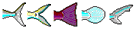Values shown below are defaults. Please double-check, replace with better values as appropriate, and 'Recalculate'.
Life History Data on Parodon nasus
Family: Parodontidae                           Scrapetooths
Max. length (Lmax): cm SL
L infinity (Linf): = cm
K: /year      Ø' =
Median Ø' value with related Linf. and K.

Growth & mortality data
to: years      Estimated from Linf and K.
Natural mortality (M):   s.e. - /year
Estimated from Linf., K and annual mean temp. = °C
Life span (approx.): years    Estimated from Linf., K and to.     Max. age & size data
Generation time: years       Estimated from Lopt, Linf., K and to.
Age at first maturity (tm): years       Estimated from Lm, Linf., K and to.
L maturity (Lm):     s.e. - cm
Estimated from Linf.        Maturity data
L max. yield (Lopt):     s.e. - cm
Length-weight: cm => (wet weight)
W = * L ^

Length-weight data
Nitrogen & protein:
 Weight (g) => whole-body nitrogen (N) (g) => whole-body crude protein (g)
Fecundity: [ no value (min.)-no value (max.) ]       Estimated as geometric mean.          Fecundity
Relative Yield per Recruit (Y'/R): Estimate Y'/R from M/K, Lc/Linf and E.
Lc= cm    E=  /year
Emsy /year    Eopt /year
Fmsy /year    Fopt /year
Exploitation: Z=
F=
E=
Estimate Z, F, E from Lc, Lmean, Linf, K, M
Lc = cm
Lmean = cm
Resilience / productivity:
Vulnerable to extinction if decline in biomass or numbers exceeds threshold over the longer of 10 years or 3 generations.
Intrinsic rate of increase (rm): /year Lr = cm
Estimated from Fmsy at Lc = length of recruitment (Lr).
Food consumption (Q/B): times the body weight per year Enter Winf, temperature, aspect ratio (A), and food type to estimate Q/B
Winf = g      Temp. = °C
A =Detrivore Herbivore Omnivore Carnivore
Estimate growth
Note: The estimates are derived from default values taken from FishBase and will thus not be appropriate for every population. You can change these values and recalculate the life history parameters.Back to SearchSpecies summary
Life History Data created by: eagbayani, 01.06.99 ,  php script by kbanasihan 06/02/2010 ,  last modified by dsantos, 09/02/2014